Question

# Given the equilibrium constants for reactions 1 and 2, determine the equilibrium constant for reaction 3....

Given the equilibrium constants for reactions 1 and 2, determine the equilibrium constant for reaction 3.

(1) 2H2S (g) + SO2(g) = 3S(s) + 2H2O(g) K=1.00 X 10^5

(2) 3SO2(g) + O2(g) = 2SO3(g) K=11.0

(3) 4H2S(g) + 2SO3(g) = 6S(g) + 4H2O(g) + O2 K=?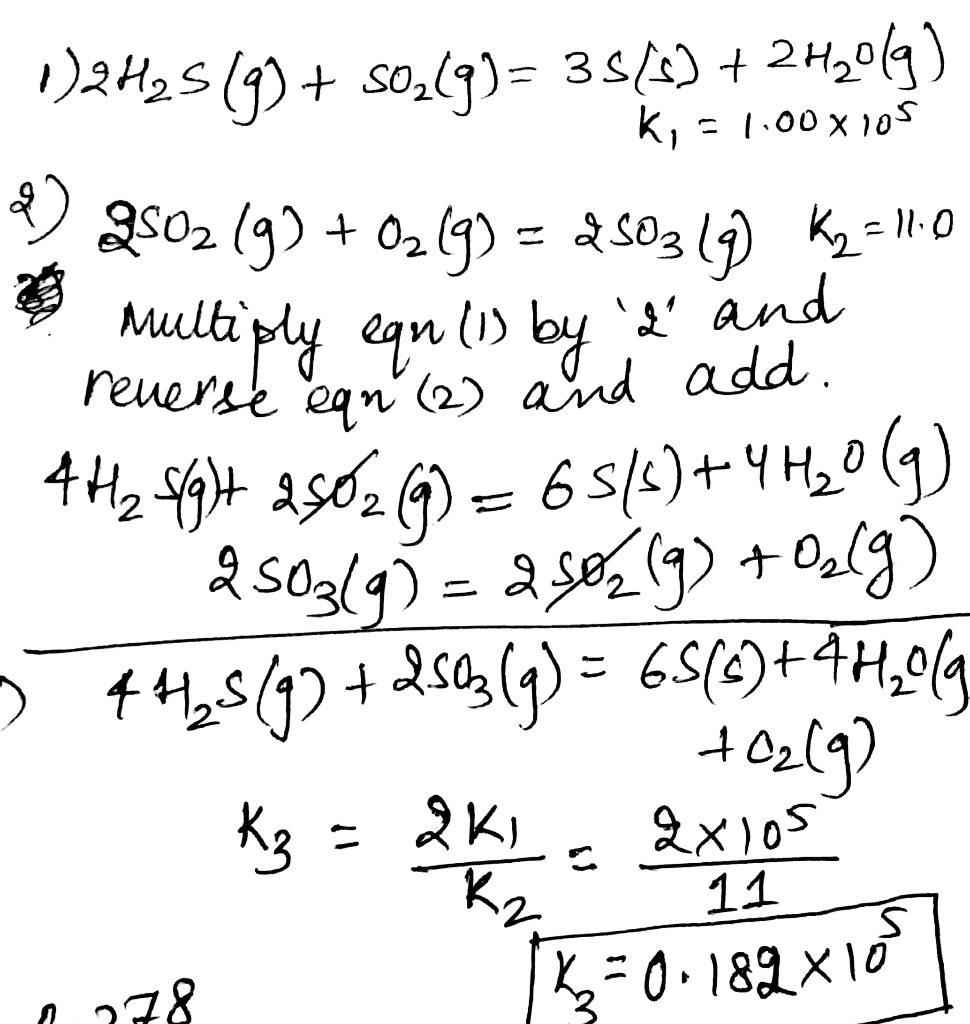#### Earn Coins

Coins can be redeemed for fabulous gifts.

Similar Homework Help Questions
• ### At a given temperature, the equilibrium constant Kc for the reaction 2SO2(g)+O2(g)<==>2SO3(g) is 2.99x10^-3.  What is the...

At a given temperature, the equilibrium constant Kc for the reaction 2SO2(g)+O2(g)<==>2SO3(g) is 2.99x10^-3.  What is the value of the equilibrium constant for each of the following reactions at that temperature? a. SO2(g)+ 1/2 O2(g)<==> SO3(g) b. 2SO3(g)<==>2SO2(g)+O2(g) c. SO3(g)<==> SO2(g)+ 1/2 O2(g)

• ### 9. (1.2 pt) At a certain temperature the following reactions have the Kc constants shown; k,...9. (1.2 pt) At a certain temperature the following reactions have the Kc constants shown; k, i = 4.2 x 1052 K. ii = 9.8 x 10128 K. = ???? → (i) S(s) + O2(g) SO2 (g) ㄹ (ii) 2 S (s) +3 O2 (g) 2SO3 (g) → Calculate the equilibrium constant Ke for the following reaction at the same temperature. 2502 (g) +02 (g) 2 SO3 (g) Kc

• ### (10) 5. Use the given reactions and their enthalpies to determine the AHºrxn for the following reaction: 2 S(x) + 3...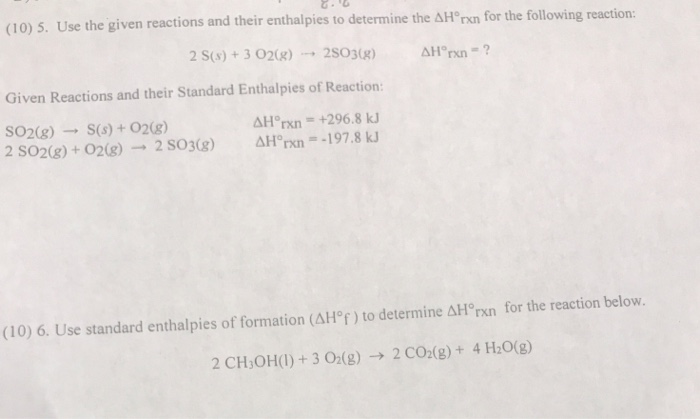(10) 5. Use the given reactions and their enthalpies to determine the AHºrxn for the following reaction: 2 S(x) + 3 O2(g) → 2503(g) Hºrxn - ? Given Reactions and their Standard Enthalpies of Reaction: SO2(g) - S(s) + O2(g) 2 SO2(g) + O2(g) 2 S03(g) AHºrxn = +296.8 kJ Hºrxn = -197.8 kJ (10) 6. Use standard enthalpies of formation (AH°f) to determine AHºrxn for the reaction below. 2 CH3OH(1) + 3 02(g) → 2 CO2(g) + 4H2O(g)

• ### 9. At a particular temperature, the following reactions have the equilibrium constants shown: S(s) + O2(g)...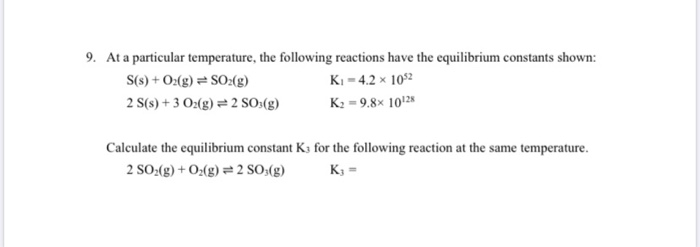9. At a particular temperature, the following reactions have the equilibrium constants shown: S(s) + O2(g) = SO2(g) K-4.2 1052 2 S(s) + 3 02(g) = 2 SO3(g) K2 = 9.8* 10128 Calculate the equilibrium constant K; for the following reaction at the same temperature. 2 SO2(g) + O2(g) = 2 SO3(g) K; =

• ### Given the equilibrium constants for the following reactions: 2H2(g) + O2(g) → 2H2O(g)    K1 =...

Given the equilibrium constants for the following reactions: 2H2(g) + O2(g) → 2H2O(g)    K1 = 3.74 x 1026 H2(g) + Cl2(g) → 2HCl(g)         K2 = 9.73 x 1012 calculate the equilibrium constant for the reaction below. 4HCl(g) + O2(g) → 2Cl2(g) + 2H2O(g)

• ### Given these equilibrium reactions: 1. % Sa(s) + 2O2te) <---------- → 2 SO2 Ka = 1.86 x 10105 2. 1/8 Ses) + 3/2 O...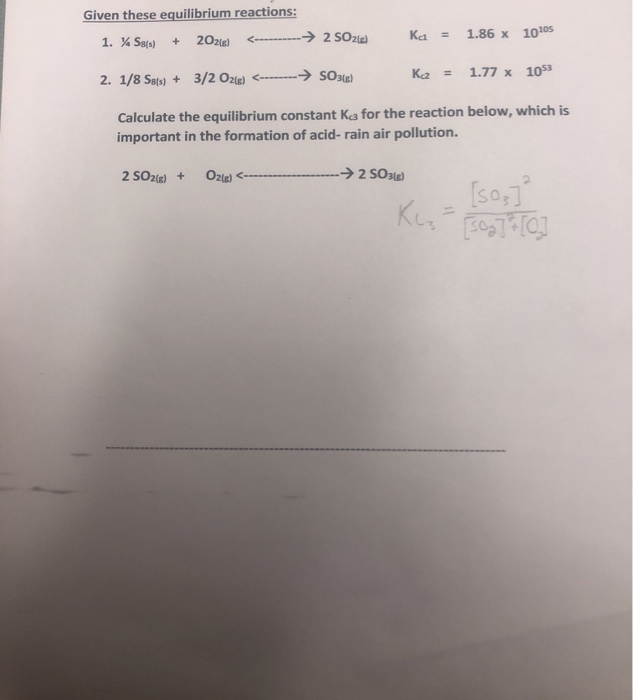Given these equilibrium reactions: 1. % Sa(s) + 2O2te) <---------- → 2 SO2 Ka = 1.86 x 10105 2. 1/8 Ses) + 3/2 O2(e) <--------→ SO3(e) ka = 1.77 x 1053 Calculate the equilibrium constant ka for the reaction below, which is important in the formation of acid-rain air pollution. 2 SO2(g) + O2(g) ------ --→2 SO3(g) [SO] Klz- 30g] 7 

• ### At 1565K, the equilibrium constant for the reactions: (1) 2H2O(g) <----> 2H2(g) +O2(g) and (2) 2CO2(g)...

At 1565K, the equilibrium constant for the reactions: (1) 2H2O(g) <----> 2H2(g) +O2(g) and (2) 2CO2(g) <----> 2CO(g) +O2(g) are 1.6*10^-11 and 1.3*10^-10, respectively. a. what is the value of the equilibrium constant for the reaction: (3) CO2(g) + H2(g) <----> H2O(g) + CO(g) at this temperature? b. demonstrate how the calculations of equilibrium constants matches the calculations of dG0r when adding two reactions or more ; determine dG0r for reactions (1) and (2) and use these values in order...

• ### Use the equilibrium constants for the following reactions at 700 °C 2SO2(g) + O2(g)  2SO3(g) K1 =...

Use the equilibrium constants for the following reactions at 700 °C 2SO2(g) + O2(g)  2SO3(g) K1 = 4.8 2NO(g) + O2(g)  2NO2(g) K2 = 16 to determine the equilibrium constant for the following reaction.         SO 3( g) + NO( g)  SO 2( g) + NO 2( g)

• ### The equilibrium constant in terms of pressures, Kp, for the reaction of SO2 and O2 to...

The equilibrium constant in terms of pressures, Kp, for the reaction of SO2 and O2 to form SO3 is 0.365 at 1.15×103 K: SO2(g) + O2(g) = 2SO3(g) A sample of SO3 is introduced into an evacuated container at 298 K and allowed to dissociate until its partial pressure reaches an equilibrium value of 0.867 atm. Calculate the equilibrium partial pressures of SO2 and O2 in the container. PSO2 = PO2 =

• ### [Refer Given the following equilibrium constants at 425°C, 1 Na2O(s) – 2 Na(1) +02(9) K= 5...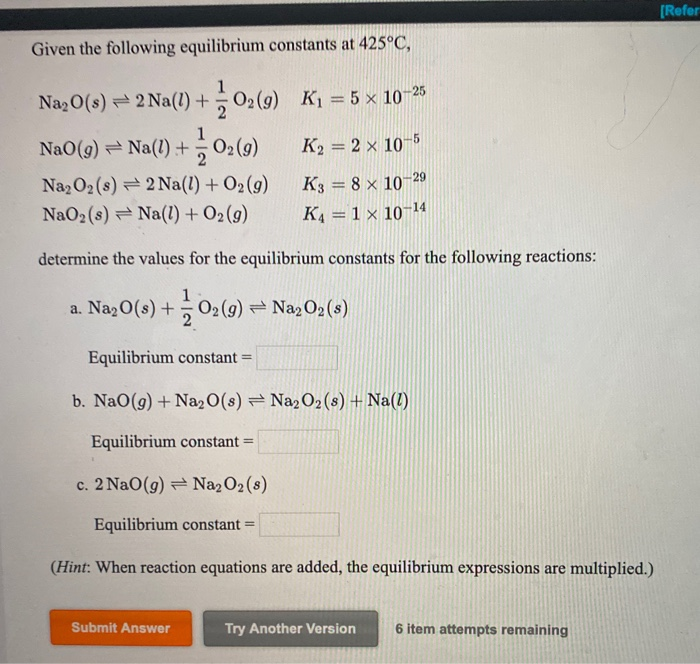[Refer Given the following equilibrium constants at 425°C, 1 Na2O(s) – 2 Na(1) +02(9) K= 5 x 10-25 NaO(9) = Na(1)+ - 02(9) K2 = 2 x 10-5 Na, O2(8) = 2 Na(l) + O2(9) K3 = 8 x 10-29 NaO2(s) = Na(l) + O2(9) K4 =1 10-14 determine the values for the equilibrium constants for the following reactions: a. Na2O(s) + + 02 (9) = Naz02 (8) Equilibrium constant = b. NaO(g) + Na2O(8) = Na2O2(8) + Na(1) Equilibrium...